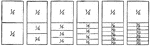### Fraction Comparisons

Rectangles comparing fraction values to one half: 1/4, 1/6, 1/8, 1/10, and 1/12.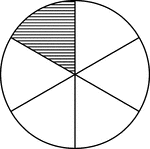### Fraction Pie Divided into Sixths

A circle divided into sixths with one sixth shaded.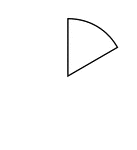### One Sixth of a Fraction Pie

One sixth of a circle.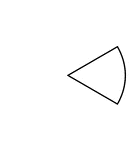### One Sixth of a Fraction Pie

One sixth of a circle.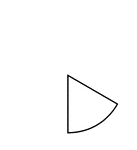### One Sixth of a Fraction Pie

One sixth of a circle.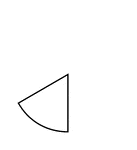### One Sixth of a Fraction Pie

One sixth of a circle.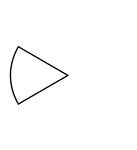### One Sixth of a Fraction Pie

One sixth of a circle.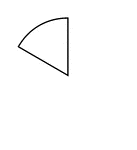### One Sixth of a Fraction Pie

One sixth of a circle.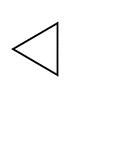### Fractions of 6-sided Polygon

1/6 of a 6 sided polygon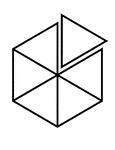### Fractions of 6-sided Polygon

6/6 of a 6 sided polygon with one piece detached